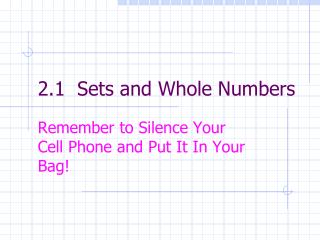Download Presentation2.1 Sets and Whole Numbers# 2.1 Sets and Whole Numbers - PowerPoint PPT Presentation

Download Presentation##### 2.1 Sets and Whole Numbers

Download Policy: Content on the Website is provided to you AS IS for your information and personal use and may not be sold / licensed / shared on other websites without getting consent from its author. While downloading, if for some reason you are not able to download a presentation, the publisher may have deleted the file from their server.

- - - - - - - - - - - - - - - - - - - - - - - - - - - E N D - - - - - - - - - - - - - - - - - - - - - - - - - - -
##### Presentation Transcript

1. 2.1 Sets and Whole Numbers Remember to Silence Your Cell Phone and Put It In Your Bag!

2. Sets • Intuitive definition of Set – A collection of objects • List the objects of a set within braces { } • When listing the objects of a set, separate them with commas • Use a capital letter to name a set • Order of the elements is not important • Element x A, x  A • A versus a

3. One-to-One Correspondence Sets A and B have one-to-one correspondence iff each element of A can be paired with exactly one element of B and each element of B can be paired with exactly one element of A.

4. Equal and Equivalent Sets • Sets A and B are equal sets, symbolized by A = B, iff each element of A is also an element of B and each element of B is also an element of A. • Sets A and B are equivalent sets, symbolized by A  B, iff there is a one-to-one correspondence between A and B.

5. Subset and Proper Subset • For all sets A and B, A is a subset of B, symbolized as A  B, iff each element of A is also an element of B. • For all sets A and B, A is a proper subset of B, symbolized by A  B, iff A is a subset of B and there is at least one element of B that is not an element of A.

6. Additional Terminology • The Universal Set U • Empty Set or Null Set  { } • Finite Set • Infinite Set

7. The Complement of a Set • The complement of set A, written Ᾱ, consists of all of the elements in U that are not in A.

8. Whole Number • A whole number is the unique characteristic embodied in each finite set and all the sets equivalent to it. • The number of elements in set A is expressed as n(A) and is called the cardinality of set A.

9. Counting Counting is the process that enables people systematically to associate a whole number with a set of objects.

10. Ordering Whole Numbers • For whole numbers a and b and sets A and B, where n(A) = a and n(B) = b, a is less than b, symbolized as a < b, iff A is equivalent to a proper subset of B. • a is greater than b, written a > b, iff b < a

11. Special Subsets of the Set of Whole Numbers • Counting Numbers or Natural Numbers {1, 2, 3, 4, . . . } • Whole Numbers {0, 1, 2, 3, . . . } • Even Whole Numbers {0, 2, 4, 6, . . . } • Odd Whole Numbers {1, 3, 5, 7, . . . }

12. Three Types of Numbers • Nominal Number • Ordinal Number • Cardinal Number

13. An announcer says: "The student with ID number 50768-973 has just won second prize--four tickets to the big game this Saturday."